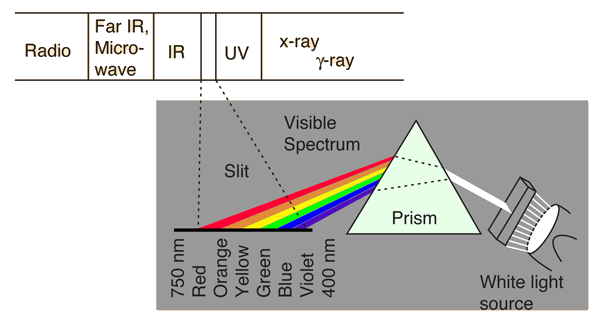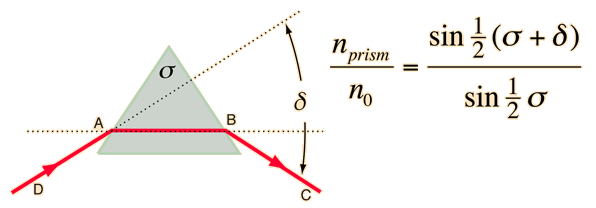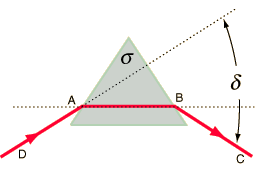# Prisms

A refracting prism is a convenient geometry to illustrate dispersion and the use of the angle of minimum deviation provides a good way to measure the index of refraction of a material. Reflecting prisms are used for erecting or otherwise changing the orientation of an image and make use of total internal reflection instead of refraction.White light may be separated into its spectral colors by dispersion in a prism.Prisms are typically characterized by their angle of minimum deviation d. This minimum deviation is achieved by adjusting the incident angle until the ray passes through theprism parallel to the bottom of the prism.

An interesting application of refraction of light in a prism occurs in atmospheric optics when tiny hexagonal ice crystals are in the air. This refraction produces the 22° halo commonly observed in northern latitudes. The fact that these ice crystals will preferentially orient themselves horizontally when falling produces a brighter part of the 22° halo horizontally to both sides of the sun; these bright spots are commonly called "sundogs".

 Calculation
 Polarizing prisms
Index

 HyperPhysics***** Light and Vision R Nave
Go Back

# Prisms

The angle of minimum deviation for a prism may be calculated from the prism equation. Note from the illustration that this minimum deviation occurs when the path of the light inside the prism is parallel to the base of the prism. If the incident light beam is rotated in either direction, the deviation of the light from its incident path caused by refraction in the prism will be greater.

White light may be separated into its spectral colors by dispersion in a prism.Unless otherwise specified, the medium will be assumed to be air. n0 =
Active formulaEnter data below and then click on the quantity you wish to calculate in the active formula above.

For a prism of apex angle
 σ = °
and index of refraction
 nprism =
the angle of minimum deviation is
 δ = °

Index

 HyperPhysics***** Light and Vision R Nave
Go Back